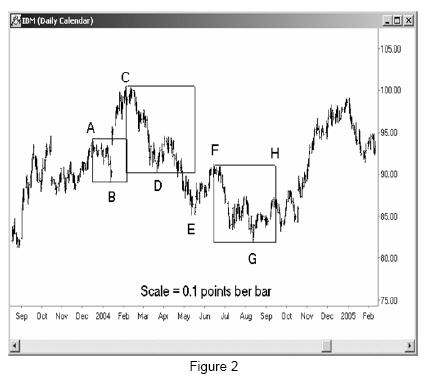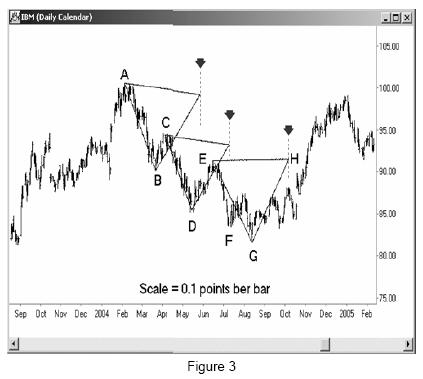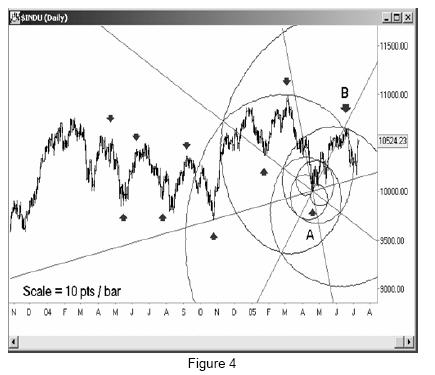# Price, Time and Market Geometry

By Earik Beann

In this article, I’d like to take a look at a subtle yet powerful relationship between time and price that is misunderstood and overlooked by 99% of traders, but that has allowed the select few who truly understand it to consistently and repeatedly pinpoint market reversals weeks and months ahead of time.

In most of the works written on technical analysis, you’ll fi nd that the charting techniques tend to fall into one of two general categories. The first category is price analysis, such as Fibonacci retracements, where the major focus of the technique is the price of the stock or commodity being analyzed. For example, Fibonacci retracements are calculated by subtracting a recent swing high and swing low to obtain a difference. Th is difference is then multiplied by various ratios, and added to or subtracted from the most recent swing level to obtain future areas of support and resistance. The focus of this tool is entirely based on the price axis of the chart, with no information given as to when a particular projection ought to take place, only the price level at which it will happen. Most oscillator style tools fall into this category as well, being calculated using inputs limited to the last few price points recorded on the chart.

The second category is time analysis. You can apply the Fibonacci techniques to the time axis as well, measuring the time between two swing points, multiplying by the appropriate ratios, and adding this distance to the last swing point to get projections of future turning points. This tool works primarily on the time axis, with no regard to the price at which the projected swing is expected to occur at. Cycle analysis falls in this category as well. While prices tend to be used in cycle analysis as a way to pin down the appropriate cycles to use, this information gets washed out in the algorithm and we are left with nothing more than time projections telling us to expect a turn at a given time but with no information about the price which this turn ought to happen at.

The point here is that we’ve all been trained to regard the time and price axes of the chart as two separate and totally independent things, and the techniques we use reflect this view. While we need both time and price values in order to construct our chart, we treat them as two separate variables, each with their own units and labels that don’t cross over. Just as you can’t convert from inches to gallons, we assume that the time and price axes of our chart are similarly segregated and independent, as the units measure two totally diff erent and separate things.

In trading, all assumptions must be questioned, especially the most basic ones. In my work, I’ve discovered that markets represent energy movement, and this energy movement expresses itself in both time and price simultaneously. What this means, from the perspective of markets, is that there really isn’t any difference between time and price aft er all. Time and price are recorded in separate units, but rather than being like inches and gallons as everyone assumes, they are more like inches and centimeters. Both measure the same energy just using different labels. This means that once we know how, we can convert between the two, transforming price into time and time into price.

This is one of the reasons why WD Gann was able to make projections in both time and price simultaneously. He didn’t just say that price would hit a specific level, or that there would be a turn at a specific date. He would say that the market would turn on a specific day at a specific price. There was both a time component and a price component to his forecasts, which is something that would have been impossible for him to do if he focused his techniques on only one axis of the chart as most traders do today.

With this idea in mind, let’s take a look at a very basic example of what we can do with this information. Please take a look at Figure 1, a daily chart of IBM.In early February of 2004, IBM made a high at 100.43 and spent the next six months in a decline. The price at the high represents a very important vibration level for this market, and like a rock thrown into a pond, it will generate ripples that move out into the future on our chart. Detecting these ripples is very easy, once we know to look for them. On the chart, I’ve taken 100.43 as a base unit, then multiplied it out by various Fibonacci ratios to obtain the following:

100.43 x 0.382 = 38.3 (0.618 x 0.618)
100.43 x 0.618 = 62.06 (0.618 = 1/golden ratio)
100.43 x 1.0 = 100.43 (the most basic ratio)
100.43 x 1.27 = 127.54 (1.27 is the square root of 1.618)
100.43 x 1.618 = 162.49 (1.618 = golden ratio)

Then, for kicks, we can also throw in a couple other ratios common in Sacred Geometry:

100.43 x 1.73 = 173.89 (root 3)
100.43 x 2.23 = 224.5 (root 5)

Now, using our high as our initial starting point, we count forward to find areas on the chart where the market should react. I counted forward using degrees of movement of the Sun, the true timekeeper. So take our high, add 62 degrees of movement of the Sun, and you get the reaction high in April. Take the high, add 100 degrees, and you get the date for the low in May.

You can see on the chart how well this technique worked to locate turning points in the future. The important thing to realize here is that we are using a pure price number to generate pure time targets. This would not be possible if there was no relationship between the time and price axes on our chart. Th is is not a selected example either – you can take any chart and repeat this exercise and you’ll see that an important price will generate important times in the future. The only requirement to do this successfully is the proper conversion factor between the two. I’ve used the Sun in this case, which works great on IBM, but diff erent markets have diff erent vibration rates, so keep that in mind when you experiment with this technique on your own charts.

Once you know the conversion factor between time and price for your market and timeframe, that factor should never change and will remain valid forever. Just as every object has a resonant frequency at which it absorbs energy, every market has a factor that it uses to convert price to time and time to price.

When you know the right factor for your market, you can set your chart up in such a way that you can actually see the relationships visually. This is called proper chart scaling or square scaling. All the legendary traders of the past like Gann, Bayer, and Marechal knew about chart scaling. If you draw charts by hand, you have to determine the scale ahead of time, and the chart scale is a direct connection between the price axis and the time axis. So if you take a look at Figure 2, you’ll find our IBM chart drawn out using the scale of 0.1 points per bar. What this means is that for every bar width on the chart, we need to go up 0.1 points on our price axis. If there are 300 bars on the chart, and our chart is perfectly square, then our price axis needs to cover exactly 30 points of vertical movement (300 x 0.1). Said another way, if our chart is 10 inches long and covers 300 days, then 30 points of price movement also needs to take up 10 inches of paper. If we have a rectangular chart, then we need to take the dimensions of the paper into account when calculating the height of our price axis. When this is all done correctly, then a 45 degree line drawn on the chart will represent a perfect balance of price movement and time movement, given the market’s built-in exchange rate between the two.A chart that is properly scaled in this way will suddenly exhibit geometrical patterns and shapes that were not visible beforehand. Th ese patterns are more than just pretty – you can use them to trade. Take a look at Figure2, where I’ve added 3 simple squares to the chart.

All I’ve done is take a swing high and a swing low, and drawn a square starting from the first point and ending at the second. So our fi rst square begins at point A, then extends down to the level of the low at point B. Find that square, then move over to the rightmost edge and you’ll see that it lines up with the time of our turn at C. Th e next square moves from C to D, and gave us a turn at E, not to mention a price level at F. The third square moved from F to G, and generated the turn at H.

I only added three squares to this chart to keep it clean and easy to see what was happening, but there are many more. Most of the highs and lows on the way down from C to G were marked off by squares based on previous swings, and it would be a great exercise for an enterprising trader to go back and locate all the squares on this chart. Note that our scale factor of 0.1 points per bar works well on most stocks, although commodities all have diff erent scales that have to be determined before this approach can be used on them.

Let’s take a look at the same chart, but this time using triangles. Please turn your attention to Figure 3.Again, we have three triangles drawn on the chart. The first triangle extends from point A to B, and if you move your eyes over to the rightmost tip, you’ll see that it lines up with the low at D. The next triangle has an edge running from C to D, and the third point coincides with the low at F. The last triangle runs from E to G, with the projected turn falling on H.

None of this is very complicated. We are using the simplest of shapes, and just drawing them in on our charts. A moving average is more difficult to calculate than this, but you’ll never see a moving average telling you anything about turning points months in advance.